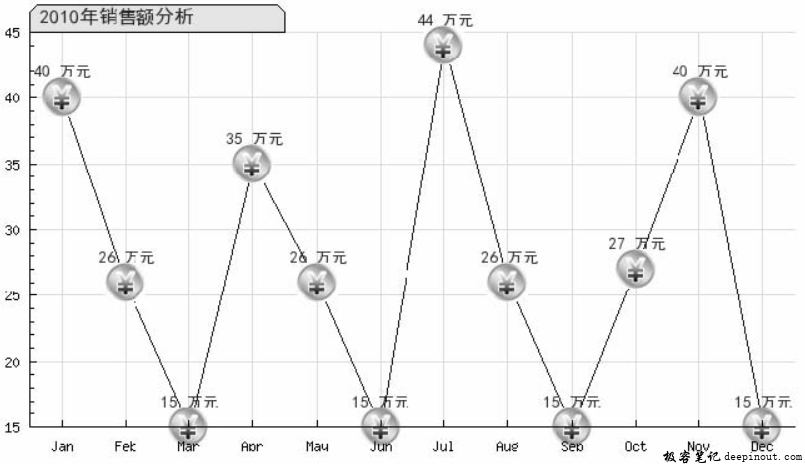# PHP 折线图绘制类

PHP 折线图绘制类——封装折线图绘制方法，绘制折线图应用的是Jpgraph类库中的LinePlot类。输出数据应用的是value()对象中的Show()方法，折线图中标记的添加应用的是mark对象的SetType()方法。

## PHP 折线图绘制类 语法

（1）使用LinePlot对象绘制曲线。

$linePlot = new LinePlot($data)       //创建折线图
$data：数值型数组，指定统计数据。  （2）输出数据应用的是value()对象中的Show()方法，数据的格式化应用的是SetFormat()方法。 SetFormat($aFormat, $aNegFormat)  •$aFormat：设置格式化的样式。

• $aNegFormat：设置negative的值。 （3）折线图中标记的添加应用的是图像中的mark对象的SetType()方法，其参数值设置标记的类型。其语法如下： SetType($aType, $aFileName,$aScale)

• $aType：设置标记的类型。 •$aFileName：插入图像的名称或者国家的名称。
• $aScale：设置插入标记的大小。 下面列举了一些SetType()方法中第一个参数可选的类型：MARK_SQUARE、MARK_UTRIANGLE、MARK_DTRIANGLE、MARK_DIAMOND、MARK_CIRCLE。 ## PHP 折线图绘制类 示例 在本示例中运用Jpgraph生成折线图，对公司2010年的销售额进行分析。 其运行结果如下图所示。折线图分析2010年公司销售额走势的关键步骤如下： （1）创建index.php文件，设置网页的编码格式，并通过include()语句导入Jpgraph类库。 （2）应用Jpgraph类库中的方法创建一个折线图，对2010年公司的销售额进行分析。其代码如下： <?php header ( "Content-type: text/html; charset=UTF-8" ); //设置文件编码格式 include ("../src/jpgraph.php"); include ("../src/jpgraph_line.php"); include ("../src/jpgraph_scatter.php");$datay1 = array(40,26,15,35,26,15,44,26,15,27,40,15);
$graph = new Graph(650,375); //定义图像大小$graph->SetMarginColor('white');                             //背景颜色
$graph->SetScale("textlin"); //定义刻度值类型$graph->SetFrame(false);
$graph->SetMargin(30,5,35,20); //设置边距$graph->tabtitle->Set(iconv("utf-8","gb2312",'2010 年销售额分析') ); //输出标题
$graph->tabtitle->SetFont(FF_SIMSUN, FS_BOLD,12); //设置标题字体$graph->tabtitle->SetColor('darkred','#E1E1FF');             //设置标题颜色
$graph->xgrid->Show(); //设置阴影$graph->xaxis->SetTickLabels($gDateLocale->GetShortMonth()); //定义X 轴上的数据$p1 = new LinePlot($datay1); //创建图像$p1->SetColor("navy");                                       //设置图像颜色
$p1->mark->SetType(MARK_IMG,'saab_95.jpg',0.5); //设置标签的样式，使用图片$p1->value->SetFormat('%d '.iconv("utf-8","gb2312",'万元')); //格式化数据
$p1->value->Show(); //输出阴影$p1->value->SetColor('darkred');                             //定义颜色
$p1->value->SetFont(FF_SIMSUN, FS_BOLD,10); //设置字体$p1->value->SetMargin(14);                                   //设置位置、字体大小
$p1->SetCenter();$graph->Add($p1); //添加数据$graph->Stroke();                                            //生成图像
?>


• 回顶